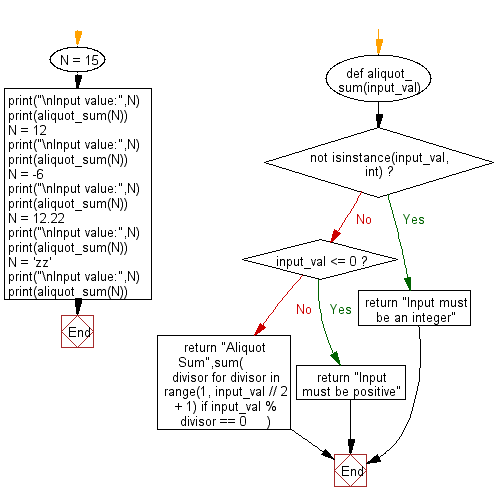﻿ Python Math: Calculate the aliquot sum of an given integer - w3resource# Python Math: Calculate the aliquot sum of an given integer

## Python Math: Exercise-83 with Solution

In number theory, the aliquot sum s(n) of a positive integer n is the sum of all proper divisors of n, that is, all divisors of n other than n itself. It can be used to characterize the prime numbers, perfect numbers, deficient numbers, abundant numbers, and untouchable numbers, and to define the aliquot sequence of a number.

For example, the proper divisors of 15 (that is, the positive divisors of 15 that are not equal to 15) are 1, 3 and 5, so the aliquot sum of 15 is 9 i.e. (1 + 3 + 5).

Write a Python program to calculate the aliquot sum of an given integer.

Sample Solution:

Python Code:

``````def aliquot_sum(input_val):
if not isinstance(input_val, int):
return "Input must be an integer"
if input_val <= 0:
return "Input must be positive"
return "Aliquot Sum",sum(
divisor for divisor in range(1, input_val // 2 + 1) if input_val % divisor == 0
)
N = 15
print("\nInput value:",N)
print(aliquot_sum(N))
N = 12
print("\nInput value:",N)
print(aliquot_sum(N))
N = -6
print("\nInput value:",N)
print(aliquot_sum(N))
N = 12.22
print("\nInput value:",N)
print(aliquot_sum(N))
N = 'zz'
print("\nInput value:",N)
print(aliquot_sum(N))
```
```

Sample Output:

```Input value: 15
('Aliquot Sum', 9)

Input value: 12
('Aliquot Sum', 16)

Input value: -6
Input must be positive

Input value: 12.22
Input must be an integer

Input value: zz
Input must be an integer
```

Flowchart:## Visualize Python code execution:

The following tool visualize what the computer is doing step-by-step as it executes the said program:

Python Code Editor:

Have another way to solve this solution? Contribute your code (and comments) through Disqus.

What is the difficulty level of this exercise?

Test your Programming skills with w3resource's quiz.

﻿

```>>> students = [{'name': 'John', 'score': 98}, {'name': 'Mike', 'score': 94}, {'name': 'Jennifer', 'score': 99}]